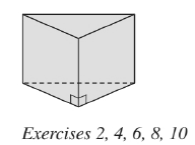Chapter 9.1, Problem 4E### Elementary Geometry for College St...

6th Edition
Daniel C. Alexander + 1 other
ISBN: 9781285195698

#### Solutions

Chapter
Section### Elementary Geometry for College St...

6th Edition
Daniel C. Alexander + 1 other
ISBN: 9781285195698
Textbook Problem
1 views

# Consider the triangular prism shown in Exercise 2. a ) How many vertices does it have? b ) How many edges (lateral edges plus base edges) does it have? c ) How many faces (lateral face plus bases) does it have?To determine

a)

To find:

The number of vertices.

Explanation

Calculation:

Given,

The triangular prism has 6 vertices...

To determine

b)

To find:

The number of edges (lateral edges plus base edges).

To determine

c)

To find:

The number of faces (lateral faces plus bases).

### Still sussing out bartleby?

Check out a sample textbook solution.

See a sample solution

#### The Solution to Your Study Problems

Bartleby provides explanations to thousands of textbook problems written by our experts, many with advanced degrees!

Get Started

#### 6. Compute .

Mathematical Applications for the Management, Life, and Social Sciences

#### Evaluate 1m when m=3.

Calculus: An Applied Approach (MindTap Course List)

#### Find for defined implicity by .

Study Guide for Stewart's Multivariable Calculus, 8th

#### True or False: A trigonometric substitution is necessary to evaluate xx2+10dx.

Study Guide for Stewart's Single Variable Calculus: Early Transcendentals, 8th